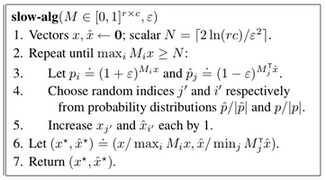## neal young / Koufogiannakis13Nearly

• Algorithmica 70(4):648-674(2014); FOCS'07We give an approximation algorithm for packing and covering linear programs (linear programs with non-negative coefficients). Given a constraint matrix with $$N$$ non-zeros, $$r$$ rows, and $$c$$ columns, the algorithm (with high probability) computes feasible primal and dual solutions whose costs are within a factor of $$1+\epsilon$$ of the optimal cost in time $$O((r+c)\log(N)/\epsilon^2 + N)$$.
Journal version of .